# C Program to Solve Linear Equations using Gauss Elimination Method

The linear equations in a matrix form are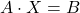and we want to find the values of X. You can solve it in many ways, and one of the simplest ways to solvesystem of equations is Gauss elimination method. It is also known as Reduction method.

This example program solves any kind of linear equation of matrix form using Gauss elimination method.

## Problem Definition

This program asks users to input the number of equations in the system of linear equations. The system of the equation looks like the following if the number of an equation is 3.

Example,

\begin{aligned}&x_1 + x_2 + x_3 = 2\\ \\
&2x_1 - 3x_2 + 2x_3 = -6 \\ \\
&x_1 + x_2 - 3x_3 = 6
\end{aligned}

The same system of equation in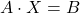form will be

\begin{bmatrix}1 & 1 & 1 \\2& -3 & 2  \\ 1&1& -3 \end{bmatrix} \cdot  \begin{bmatrix}x_1 \\ x_2\\ x_3\end{bmatrix} = \begin{bmatrix}2\\-6\\6\end{bmatrix}

You want to find the solution to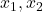and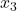using the Gauss elimination method. The Gauss elimination method is done using a series of row and column operations on the coefficient matrix. The coefficient matrix must be a square matrix otherwise the equation will not work.

For example, if we perform a series of row operation on the above matrix.

R2 -> R2 - 2R1
R3 -> R3 - R1

where R2 is row2 and R3 is row3.

You get an upper triangular matrix as shown below. The equation is in the form.

\begin{bmatrix}1 & 1 & 1 \\0&-5&0\\0&0& -4 \end{bmatrix} \cdot  \begin{bmatrix}x_1 \\ x_2\\ x_3\end{bmatrix} = \begin{bmatrix}2\\-10\\4\end{bmatrix}

Note that whatever operations performed on A will also be performed on B. Finally, by “back substitution”, you can find values of other solutions.

for example,

 \begin{aligned}&-5x_2 = -10\\
& x_2 = 2\\\\
&x_1 + 2 - 1 = 2\\
&x_1 =1\\\\
&-4x_3=4\\
&x_3=-1\end{aligned}

Now we have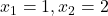and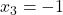as solution the system of equations.

## Program Code

/* Program to solve the given system of equations

x1 - x2 + x3 = 1
-3x1 + 2x2 - 3x3 = -6
2x1 - 5x2 + 4x3 = 5

We will use Gauss Elimination Method to solve it */

#include <stdio.h>
#include <stdlib.h>
#include <math.h>

int main()
{

int n,i,j,k;
float a={0.0}, x={0.0};
float pivot = 0.0;
float factor = 0.0;
float sum = 0.0;
printf("Solution by Simple Gauss Elimination \n\n");

printf("How many elements of the equations:");

scanf("%d",&n);

printf("%d\n",n);

printf("\n\t Input Coefficients a[i, j+1], row-wise\n");

for(i=1;i<=n;i++)
{

for(j=1;j<=n+1;j++)
{
scanf("%f",&a[i][j]);
}

}
printf("\n\n");
for(i=1;i<=n;i++)
{
for(j=1;j<=n+1;j++)
{

printf("\t%10.0f",a[i][j]);

}

printf("\n\n");

}

for(k=1;k<=n-1;k++)
{

if(a[k][k]==0.0)
{

printf("error");

}
else
{
pivot = a[k][k];
for(j=k;j<=n+1;j++)
a[k][j]= a[k][j]/pivot;

for(i=k+1;i<=n;i++)
{
factor = a[i][k];

for(j = k;j<=n+1;j++)
{
a[i][j] = a[i][j] - factor * a[k][j];
}
}

}

if(a[n][n]==0)

printf("error");

else
{

x[n] = a[n][n+1]/a[n][n];

for(i=n-1;i>=1;i--)
{

sum = 0.0;

for(j=i+1;j<=n;j++)

sum = sum + a[i][j] * x[j];

x[i]= ( a[i][n+1]- sum )/a[i][i];

}

}

}

for(i=1;i<=n;i++)
{

printf("\n\tx[%1d]=%10.4f",i,x[i]);

}

system("PAUSE");
return 0;

}

## Output

   2             1             1            1

-6             2            -3            2

6             1             1           -3

x=     1.0000
x=     2.0000
x=    -1.0000
Press any key to continue . . .